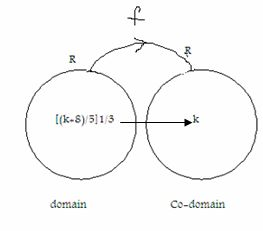Email us to get an instant 20% discount on highly effective K-12 Math & English kwizNET Programs!

#### Online Quiz (WorksheetABCD)

Questions Per Quiz = 2 4 6 8 10

### High School Mathematics - 24.4 Many-one, One-One Functions

 Many - One Function: If the function f: A->B is such that 2 or more elements a1, a2.... of A have the same f-image in B, then the mapping is called many-one mapping or many-one function.Note that f:A->B is not one-one then it is many-one.One - One Onto Function If the function f is both one-one and onto then f is called one-one onto function. It is also called a bijection or a bijective function.In other words, a function f: A ->B is bijective if if it is one-one, i.e f(x) = f(y) which implies x = y for all x,y € A it is onto,i.e, for all y € B, there exists x € A such that f(x) = y. Example: If R->R be a function defined by f(x) = 5x3-8, show that the function is a bijective function. Solution: f(x) = 5x3-8 , x € R be any two elements of R (domain) f is one-one (injective) Let x,y €R then f(x) = f(y) which implies 5x3 - 8 = 5y3 - 8 From the above we get x3 = y3 Hence x3 = y3 = 0 (x-y) = 0, because the second factor is not equal to zero. x = y Hence is one-one. f is onto, i.e surjective. Let k be any real number in the codomain. Then f(x) = k = 5x3-8 k => x = {(k+8)/5}1/3 Now, f[(k+8)/5]1/3 Directions: Answer the following.
 Q 1: If A = {1,2,3}, B = {4,5) and f = {(1,4), (1,5),(2,4), (3,5). Is f a function from A into B?yesno Q 2: Find the range of the function [(x, (x2-1)/(x-1): x € R, x not equal to 1}R - {1}R - {2}not a function Q 3: For a function f: A->B an onto function, in this case n(B) ___ n(A)less thanmore thanless than or equal to Q 4: Is the relation given below a function?{(2,1), (5,1), (8,1), (11,1), (14,1), (17,1)}NoYes Q 5: Let f: A->B be one-to-one function such that range of f is {b}. Determine the number of elements in A.10such a function cannot exist Q 6: f(x) = x3, A function R->R, is onto or not.noyes Q 7: f(x) = x+1, A function R->R, is onto or not.noyes Q 8: {(1,2), (2,2), (3,2)}. find the rangenot a function{2}{1,2,3} Question 9: This question is available to subscribers only! Question 10: This question is available to subscribers only!

#### Subscription to kwizNET Learning System offers the following benefits:

• Unrestricted access to grade appropriate lessons, quizzes, & printable worksheets
• Instant scoring of online quizzes
• Progress tracking and award certificates to keep your student motivated
• Unlimited practice with auto-generated 'WIZ MATH' quizzes